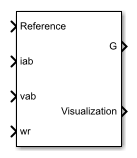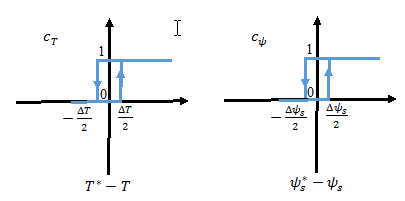# Induction Machine Direct Torque Control (Single-Phase)

Single-phase induction machine direct torque control

•Libraries:
Simscape / Electrical / Control / Induction Machine Control

## Description

The Induction Machine Direct Torque Control (Single-Phase) block implements a single-phase induction machine direct torque control structure.

### Equations

This diagram shows the direct torque control architecture for single-phase machinesThe torque and flux estimation is based on machine voltage equations. The discrete-time voltage equations using the backward Euler discretization method, are:

`${\psi }_{a}=\left({v}_{a}-{i}_{a}{R}_{as}\right)\frac{{T}_{s}z}{z-1}$`

`${\psi }_{b}=\left({v}_{b}-{i}_{b}{R}_{bs}\right)\frac{{T}_{s}z}{z-1}$`

where:

• Ras and Rbs are the main winding resistance and the auxiliary winding resistance, respectively.

• ia and ib are the main winding current and the auxiliary winding current, respectively.

• va and vb are the main and auxiliary winding voltage, respectively.

• ψa and ψb are the main and auxiliary winding flux, respectively.

The torque and flux are obtained from:

`$T=-p\left(a{\psi }_{a}{i}_{b}-\frac{1}{a}{\psi }_{b}{i}_{a}\right)$`

`${\psi }_{s}=\sqrt{{\psi }_{a}{}^{2}+{\psi }_{b}{}^{2}}$`

where:

• `p` is the number of pole pairs.

• `a` is the auxiliary to main windings turn ratio.

Employing simple hysteresis comparators detects the status of flux and torque errors. The following figures illustrate the hysteresis comparators and the switching sectors.The table shows the optimum switching table (inverter high side).

cψ, cT, S(θ)S0S1S2S3
`cψ` = 1`cT` = 11, 01, 10, 10, 0
`cT` = 01, 10, 10, 01, 0
`cψ` = 0`cT` = 10, 01, 01, 10, 1
`cT` = 00, 10, 01, 01, 1

The torque reference can be provided as an input, or, in the case of speed control, be generated internally using a PI speed controller.

The flux reference is generated internally using:

`${\psi }_{s}{}^{*}=\frac{2\pi {f}_{n}{\psi }_{n}}{p\mathrm{min}\left(|{\omega }_{r}|,\frac{2\pi {f}_{n}}{p}\right)}$`

where,

• ωr is the rotor angular mechanical speed in rad/s.

• fn is the rated frequency.

• ψn is the rated flux.

### Limitations

• The power inverter dead-times are not considered in this block. For hardware implementation, add the dead-time externally.

## Ports

### Input

expand all

Specify the angular velocity reference in rad/s or the torque reference in Nm.

Data Types: `single` | `double`

Stator currents for main and auxiliary windings.

Data Types: `single` | `double`

Stator voltages for main and auxiliary windings.

Data Types: `single` | `double`

Measure rotor angular speed in rad/s.

Data Types: `single` | `double`

### Output

expand all

Inverter gate pulses, specified as a Boolean. The dead-time is not considered.

Data Types: `single` | `double`

Bus containing internal signals for visualization. The signals are:

• Reference torque or speed

• Torque Reference, in Nm

• Flux Reference, in Wb

• Torque, in Nm

• Flux, in Wb

Data Types: `single` | `double` | `bus`

## Parameters

expand all

### General

Specify the control mode. The reference input is taken as angular speed, in rad/s, for `Speed control` and torque, in Nm, for `Torque control`.

Rated electrical frequency, in Hz.

Number of pole pairs.

Resistance of the main winding stator, in Ohms.

Resistance of the auxiliary winding stator, in Ohms.

Auxiliary-to-main windings turns ratio.

Time, in s, between consecutive block executions. During execution, the block produces outputs and, if appropriate, updates its internal state. For more information, see What Is Sample Time? and Specify Sample Time.

If this block is inside a triggered subsystem, inherit the sample time by setting this parameter to `-1`. If this block is in a continuous variable-step model, specify the sample time explicitly using a positive scalar.

### Outer Loop

This table shows how the visibility of some parameters in the Outer Loop tab depends on the option that you choose for the Control mode parameter in the General tab.

Control modeAvailable Parameters
Speed controlSpeed controller proportional gain
Speed controller integral gain
Speed controller integral anti-windup gain
Maximum torque (Nm)
Minimum torque (Nm)
Rated flux (Wb)
Torque controlRated flux (Wb)

Proportional gain for the PI speed controller.

Integral gain for the PI speed controller.

Integral anti-windup gain.

Maximum torque used in speed controller saturation, in Nm.

Minimum torque used in speed controller saturation, in Nm.

Rated flux, in Wb. The rated flux is used to compute the flux reference.

### Inner Loop

Flux hysteresis band for the controller, in Wb.

Torque hysteresis band for the controller, in Nm.

 Takahashi, I., and T. Noguchi. "A New Quick-Response and High-Efficiency Control Strategy of an Induction Motor." IEEE Transactions on Industry Applications. Vol. IA-22, Number 5, 1986, pp. 820 - 827.